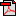# Multimedia Signal Processing Laboratory

## Paper Abstracts 1986

### Papers"The Computation of Line Spectral Frequencies Using Chebyshev Polynomials", IEEE Trans. Acoustics, Speech, Signal Processing, vol. 34, no. 6, pp. 1419-1426, Dec. 1986.

Line spectral frequencies provide an alternate parameterization of the analysis and synthesis filters used in linear predictive coding (LPC) of speech. In this paper, a new method of converting between the direct form predictor coefficients and line spectral frequencies is presented. The system polynomial for the analysis filter is converted to two even-order symmetric polynomial with interlacing roots on the unit circle. The line spectral frequencies are given by the positions of the roots of these two auxiliary polynomials. The response of each of these polynomials on the unit circle is expressed as a series expansion in Chebyshev polynomials. The line spectral frequencies are found using an iterative root finding algorithm which searches for real roots of a real function. The algorithm developed is simple in structure and is designed to constrain the maximum number of evaluations of the series expansions. The method is highly accurate and can be used in a form that avoids the storage of trigonometric tables or the computation of trigonometric functions. The reconversion of line spectral frequencies to predictor coefficients uses an efficient algorithm derived by expressing the root factors as an expansion in Chebyshev polynomials.

### Conference papers"Tree Coding in a Code Excited Linear Predictive Speech Encoder", Proc. Biennial Symp. Commun. (Kingston, ON), pp. C.3.8-C.3.11, June 1986.

Delayed decision coding together with noise masking theory is used in a code excited linear predictive speech coding system. The quantizer design is based on a modified (M,L)-algorithm which implements a multi-path tree encoder. Prediction errors are coded in blocks of N samples. The performance of the coding system as a function of M, L, N, and an additional parameter is given. High objective and subjective performance is obtained at low bit rates."The Stability of Pitch Filters in Speech Coding", Proc. Biennial Symp. Commun. (Kingston, ON), pp. C.3.4-C.3.7, June 1986.

The stability and performance of pitch filters in speech coding are studied. A new algorithm that estimates the pitch period is coupled with the covariance formulation of determining the predictor coefficients. Since this approach does not guarantee the stability of the pitch synthesis filter, an efficient stability test is formulated. From this, a stabilization technique that ensures a stable pitch filter is introduced. The effect of the presence of unstable pitch filters on decoded speech is investigated. The use of stable pitch filters in speech coding generates decoded speech of higher perceptual quality."Performance of Fixed-Point FFT's: Rounding and Scaling Considerations", Proc. IEEE Int. Conf. Acoustics, Speech, Signal Processing (Tokyo), pp. 221-224, April 1986.

The calculation of the discrete Fourier transform using a fast Fourier transform (FFT) algorithm with fixed-point arithmetic is considered. The input data is scaled to prevent overflow and to maintain accuracy. The implementation uses 16-bit fixed-point representation for the data and provides for double precision accumulation of sums and products. Algorithm variants as well as different rounding options are compared. Execution times for implementations based on a single chip signal processor are given. These show that a considerable increase in accuracy can be obtained with only a small penalty in execution time, by applying an alternating form of rounding rather than truncation.

Paper titles.